# Algebra 1 : How to multiply monomial quotients

## Example Questions

### Example Question #31 : How To Multiply Monomial Quotients

Multiply the following monomial quotients: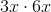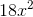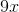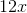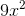Explanation:

To solve this problem, split it into two steps:

1. Multiply the coefficients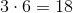2. Multiply the variables. We also need to remember the following laws of exponents rule: When multiplying variables, add the exponents.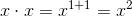Combine these to get the final answer:### Example Question #32 : How To Multiply Monomial Quotients

Multiply the following monomial quotients: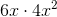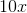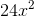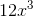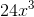Explanation:

To solve this problem, split it into two steps:

1. Multiply the coefficients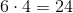2. Multiply the variables. We also need to remember the following laws of exponents rule: When multiplying variables, add the exponents.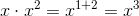Combine these to get the final answer:### Example Question #33 : How To Multiply Monomial Quotients

Multiply the following monomial quotients: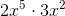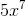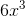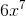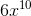Explanation:

To solve this problem, split it into two steps:

1. Multiply the coefficients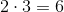2. Multiply the variables. We also need to remember the following laws of exponents rule: When multiplying variables, add the exponents.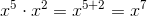Combine these to get the final answer:### Example Question #141 : Monomials

Multiply the following monomial quotiemts: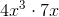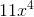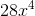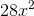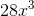Explanation:

To solve this problem, split it into two steps:

1. Multiply the coefficients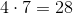2. Multiply the variables. We also need to remember the following laws of exponents rule: When multiplying variables, add the exponents.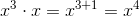Combine these to get the final answer:### Example Question #142 : Monomials

Multiply the following monomial quotients: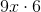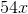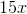Explanation:

To solve this problem, split it into two steps:

1. Multiply the coefficients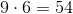2. Combine this with the lone variable to get the final answer:### Example Question #143 : Monomials

Multiply the following monomial quotients: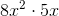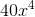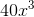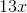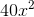Explanation:

To solve this problem, split it into two steps:

1. Multiply the coefficients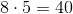2. Multiply the variables. We also need to remember the following laws of exponents rule: When multiplying variables, add the exponents.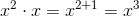Combine these to get the final answer:### Example Question #144 : Monomials

Multiply the following monomial quotients: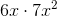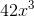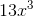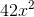Explanation:

To solve this problem, split it into two steps:

1. Multiply the coefficients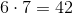2. Multiply the variables. We also need to remember the following laws of exponents rule: When multiplying variables, add the exponents.Combine these to get the final answer:### Example Question #145 : Monomials

Multiply the following monomial quotients: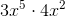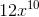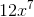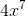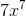Explanation:

To solve this problem, split it into two steps:

1. Multiply the coefficients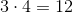2. Multiply the variables. We also need to remember the following laws of exponents rule: When multiplying variables, add the exponents.Combine these to get the final answer:### Example Question #34 : How To Multiply Monomial Quotients

Multiply the following monomial quotients: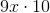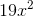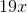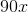Explanation:

To solve this problem, split it into two steps:

1. Multiply the coefficients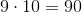2. Combine this with the lone variable e to get the final answer:### Example Question #147 : Monomials

Multiply the following monomial quotients: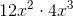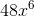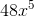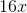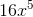Explanation:

To solve this problem, split it into two steps:

1. Multiply the coefficients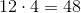2. Multiply the variables. We also need to remember the following laws of exponents rule: When multiplying variables, add the exponents.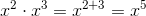Combine these to get the final answer: